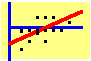8.5 Arbitrage Pricing Theory

You have already seen that a fundamental principle of valuation is that investors are compensated (in terms of expected return) for assuming risk that cannot be diversified away in the capital markets, but that they are not compensated for assuming diversifiable risk.

In Arbitrage Pricing Theory, this principle is the starting point of the theory. Let us assume that there exists a set of economic factors, unexpected changes in which cannot be eliminated through diversification. A study by Chen, Roll, and Ross (1986) argues that there are at least four such factors:

1. The level of production activity in the economy.

2. Inflationary expectations.

3. The spread between the short and long end of the default free yield curve.

4. The spread between low and high default rates on risky debt.

Now suppose that the value of your portfolio of stocks is exposed to unexpected changes in these factors. If you cannot hedge against these unexpected changes, then it is reasonable to assume that you would like to be compensated for assuming these risks.

In the APT, systematic risk is captured by economic "factors," such as the four listed above. In fact, the main assumption underlying the arbitrage pricing theory (APT) is that security returns are generated by a multi-factor model. Furthermore, it is assumed that the return-generating process model is linear, with the form: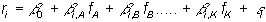where

riº the rate of return on security i, a random variable;

bi,k º the ith security's return responsiveness to factor k;

fA, º non-diversifiable factor A;

fB, º non-diversifiable factor B, and so on;

ei º the idiosyncratic risk or residual term, which is independent across securities.

The factors are assumed to be independent of each other, and of the residual term. It is also assumed that the expected value of each factor, and of the residual term, is zero; this implies that b0 = E(ri). Finally, the residual terms across firms are assumed to be independent.

Arbitrage Portfolios

Given the return-generating process, the APT derives relationships between security returns relying only on arbitrage arguments. An arbitrage portfolio is a portfolio that requires zero wealth and eliminates all unsystematic and systematic risk. The basic principle is that such a portfolio must also have zero return; otherwise, it offers an arbitrage opportunity.

To create an arbitrage portfolio, we must eliminate all systematic and unsystematic risk. Let wi = change in the share or weight of security i in our portfolio, and suppose there are n securities.

Unsystematic risk is eliminated simply by having many securities in the portfolio, and keeping the net share of any security small. In particular, we want wi to be equal to approximately 1/n. You may recall from Chapter 3, Naive Diversification, that the effects of unsystematic risk are eliminated in a large portfolio with weights equal to 1/n because in the limit, as n approaches infinity, the sum of the idiosyncratic risk terms approaches zero. To achieve this, we must have a large number of securities available.

The next requirement is that the arbitrage portfolio be a "zero wealth" portfolio. That is, from your existing position you can construct this portfolio without contributing or withdrawing any cash. This is achieved by taking both long and short positions that offset each other in costs and revenues. Of course, this requires you to be able to short-sell. The zero-wealth condition is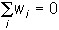The last requirement is that we eliminate all sources of systematic risk. This means that we have to choose the wi so that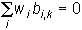for all factors k.

Arbitrage Free Prices

Together, the restrictions on the wi lead to a linear risk-return relation for any security, similar to the CAPM. In fact, the expected return for each firm can be expressed as a linear equation of K responsiveness factors plus the risk-free factor. The restrictions, together with the requirement that arbitrage portfolio's have a zero return, imply that there exist K numbers, jA,...,jK, such that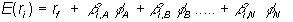This equation is frequently transformed into the "risk premium multiple regression form"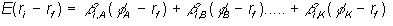The terms in the parentheses can be interpreted as the price for each component of systematic risk. Finally, in this form the beta for any factor, j, is defined as the covariance between the security's return and factor j's return scaled by the variance of factor j's return.

Relationship between CAPM and APT

How does this theory relate to CAPM theory, which by now you have become so closely acquainted with? Clearly, the APT with one factor looks a lot like the SML equation from the CAPM. Even in this simplified form, some interesting differences exist:

One factor APT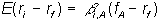SML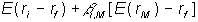Comparison of the SML with the One Factor APT

In both cases, there is a simple linear relationship between expected excess returns and a security's beta. The difference between the two models lies in the interpretation of the factor. For CAPM, this is the market index M (the value-weighted index of all risky securities). For APT this could be M, but is not restricted to M. For example, the factor could be a proxy for M. That is, APT still makes a prediction given some proxy for M something that CAPM cannot provide.

In this sense, the one-factor APT is a generalization of the CAPM. The CAPM, however, imposes less restrictive assumptions on the underlying return-generating process by not requiring it to be linear. On the other hand, the APT does not make any (restrictive) assumptions on investor preferences.

In the next topic we apply the APT to the problem of calculating optimal portfolios.

previous topic

next topic